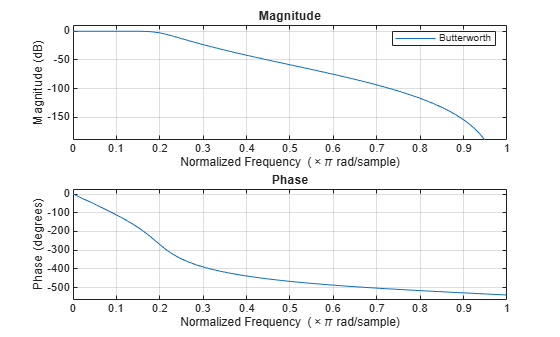# stmcb

Compute linear model using Steiglitz-McBride iteration

## Syntax

``[b,a] = stmcb(h,nb,na)``
``[b,a] = stmcb(h,nb,na,niter)``
``[b,a] = stmcb(h,nb,na,niter,ai)``
``[b,a] = stmcb(y,x,___)``

## Description

example

````[b,a] = stmcb(h,nb,na)` finds the coefficients `b` and `a` of the system b(z)/a(z) with approximate impulse response `h`, exactly `nb` zeros, and exactly `na` poles.```
````[b,a] = stmcb(h,nb,na,niter)` uses `niter` iterations. The default number of iterations is 5.```
````[b,a] = stmcb(h,nb,na,niter,ai)` uses the vector `ai` as the initial estimate of the denominator coefficients.```
````[b,a] = stmcb(y,x,___)` finds the coefficients with system output `y` and input `x` replacing `h`. `y` and `x` must be the same length.```

## Examples

collapse all

Approximate the impulse response of an IIR filter with a system of a lower order.

Specify a 6th-order Butterworth filter with normalized 3-dB frequency of $0.2\pi$ rad/sample.

```d = designfilt('lowpassiir','FilterOrder',6, ... 'HalfPowerFrequency',0.2,'DesignMethod','butter');```

Use the Steiglitz-McBride iteration to approximate the filter with a 4th-order system.

```h = impz(d); [bb,aa] = stmcb(h,4,4);```

Plot the frequency responses of the two systems.

```hfvt = fvtool(d,bb,aa,'Analysis','freq'); legend(hfvt,'Butterworth','Steiglitz-McBride')```## Input Arguments

collapse all

Impulse response, specified as a vector.

Data Types: `single` | `double`
Complex Number Support: Yes

Numerator and denominator orders, specified as positive integer scalars.

• If you want an all-pole transfer function, specify `nb` as `0`.

• If you want an all-zero transfer function, specify `na` as `0`.

Data Types: `single` | `double`

Number of iterations, specified as a positive scalar.

Initial estimate of denominator coefficients, specified as a vector. If not specified, the `stmcb` function uses the output of `prony` with the order of the numerator set to `0`.

Data Types: `single` | `double`
Complex Number Support: Yes

Output signal of the system, specified as a vector.

Data Types: `single` | `double`
Complex Number Support: Yes

Input signal of the system, specified as a vector.

Data Types: `single` | `double`
Complex Number Support: Yes

## Output Arguments

collapse all

IIR filter coefficients, returned as row vectors. `b` has length `nb` + 1 and `a` has length `na` + 1. The filter coefficients are ordered in descending powers of z.

`$H\left(z\right)=\frac{B\left(z\right)}{A\left(z\right)}=\frac{b\left(1\right)+b\left(2\right){z}^{-1}+\cdots +b\left(nb+1\right){z}^{-nb}}{a\left(1\right)+a\left(2\right){z}^{-1}+\cdots +a\left(na+1\right){z}^{-na}}$`

## Algorithms

The `stmcb` function attempts to minimize the squared error between the impulse response h of b(z)/a(z) and the input signal x.

`$\underset{a,b}{\mathrm{min}}\sum _{i=0}^{\infty }|x\left(i\right)-h\left(i\right){|}^{2}$`

The function iterates using two steps:

1. It prefilters `h` and `x` using 1/a(z).

2. It solves a system of linear equations for `b` and `a` using \.

The function repeats this process `niter` times. The function does not check to see if the `b` and `a` coefficients have converged in fewer than `niter` iterations.

 Steiglitz, K., and L. McBride. “A Technique for the Identification of Linear Systems.” IEEE® Transactions on Automatic Control 10, no. 4 (October 1965): 461–64. https://doi.org/10.1109/TAC.1965.1098181.

 Ljung, Lennart. System Identification: Theory for the User. 2nd ed. Prentice Hall Information and System Sciences Series. Upper Saddle River, NJ: Prentice Hall PTR, 1999.